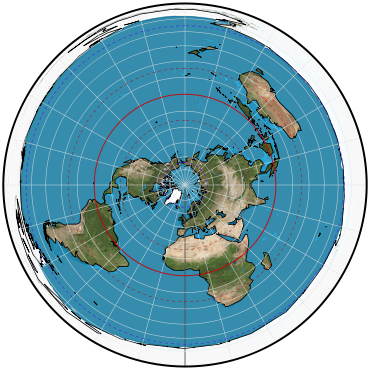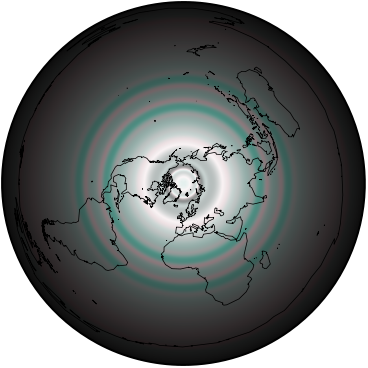## Directory of Map Projections

What is a projection?

Previous | Next

azimuthal extruded globeParameters: Radius of 85° parallel (any scale, any units), Radius of 80° parallel (scale & units of 85°), Radius of 75° parallel (scale & units of 85°), Radius of 70° parallel (scale & units of 85°), Radius of 65° parallel (scale & units of 85°), Radius of 60° parallel (scale & units of 85°), Radius of 55° parallel (scale & units of 85°), Radius of 50° parallel (scale & units of 85°), Radius of 45° parallel (scale & units of 85°), Radius of 40° parallel (scale & units of 85°), Radius of 35° parallel (scale & units of 85°), Radius of 30° parallel (scale & units of 85°), Radius of 25° parallel (scale & units of 85°), Radius of 20° parallel (scale & units of 85°), Radius of 15° parallel (scale & units of 85°), Radius of 10° parallel (scale & units of 85°), Radius of 5° parallel (scale & units of 85°), Radius of 0° parallel (scale & units of 85°)

Description

Projection for globes that are made by printing onto a plastic sheet that is then softened and extruded around a hemispherical form. In order for the resulting globe to have little distortion, the projection must invert the distortion that happens when extruding.

Classifications

azimuthal

Graticule

Meridians: Straight lines radiating from the center pole, equally spaced.
Parallels: Concentric circles spaced according to parameterization.
Poles: Center pole is a point; opposite pole is a circle—but normally only a hemisphere would be used.

Parameters

Measurement values for each parallel placement in 5° increments.

Origin

Developed Daniel “daan” Strebe, circa 1995.

Description ©2010–2020 Mapthematics LLC.